12122

# 前言

## 一定时间无操作时播放视频

``````let minute = 10
``````

``````setInterval(() => {
minute--
if (minute <= 0) {
// 播放视频
}
}, 1000 * 60)
``````

``````window.addEventListener('click', () => minute = 10)
``````

## Vue 性能优化

``````computed: {
a () { return 1 },
b ({ a }) {
return a + 10
}
}
``````

## 纯 CSS 实现拖拽效果

``````.dragbox {
width: 300px;
height: 300px;
overflow: auto
}
.dragcon {
width: 500px;
height: 500px;
}
``````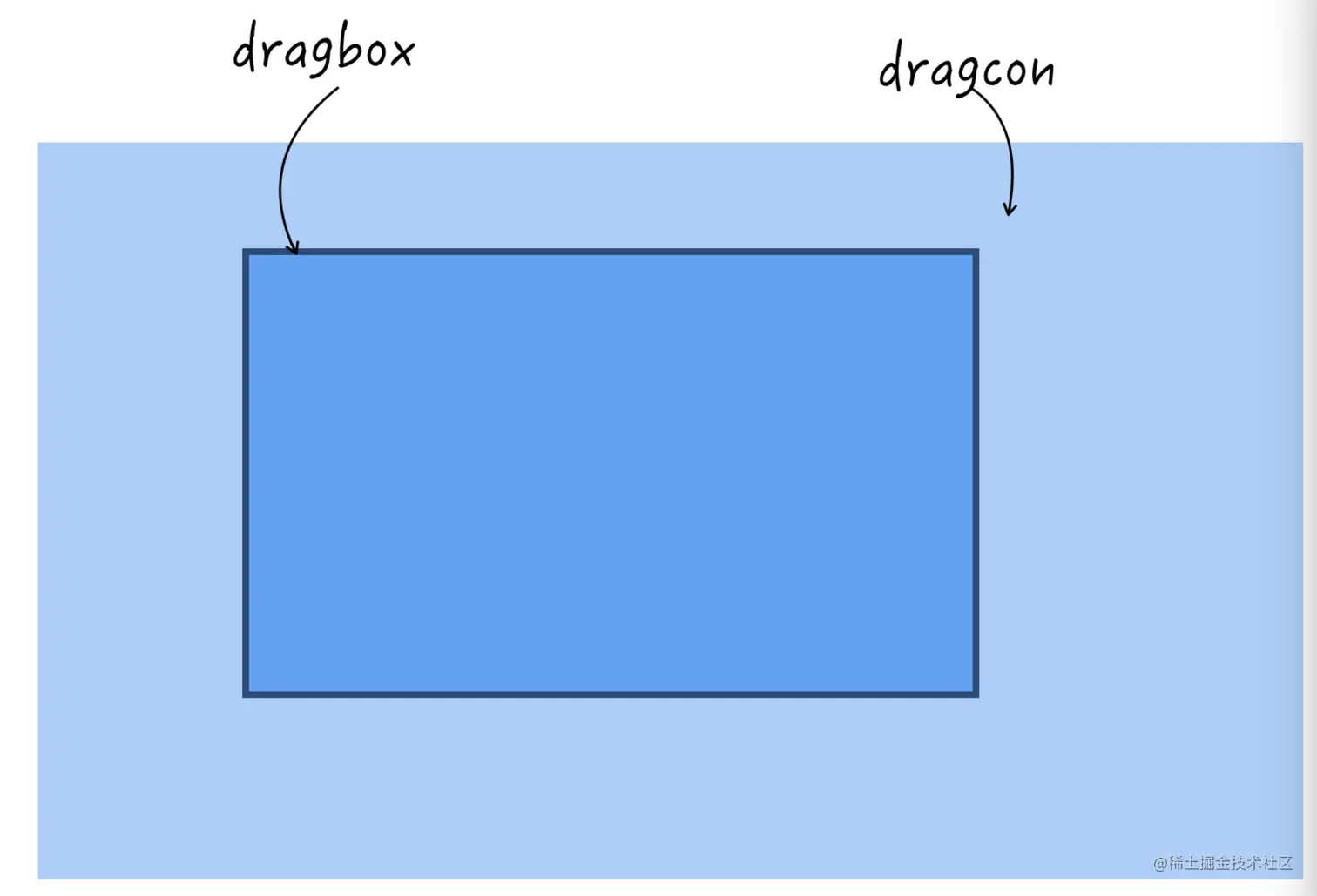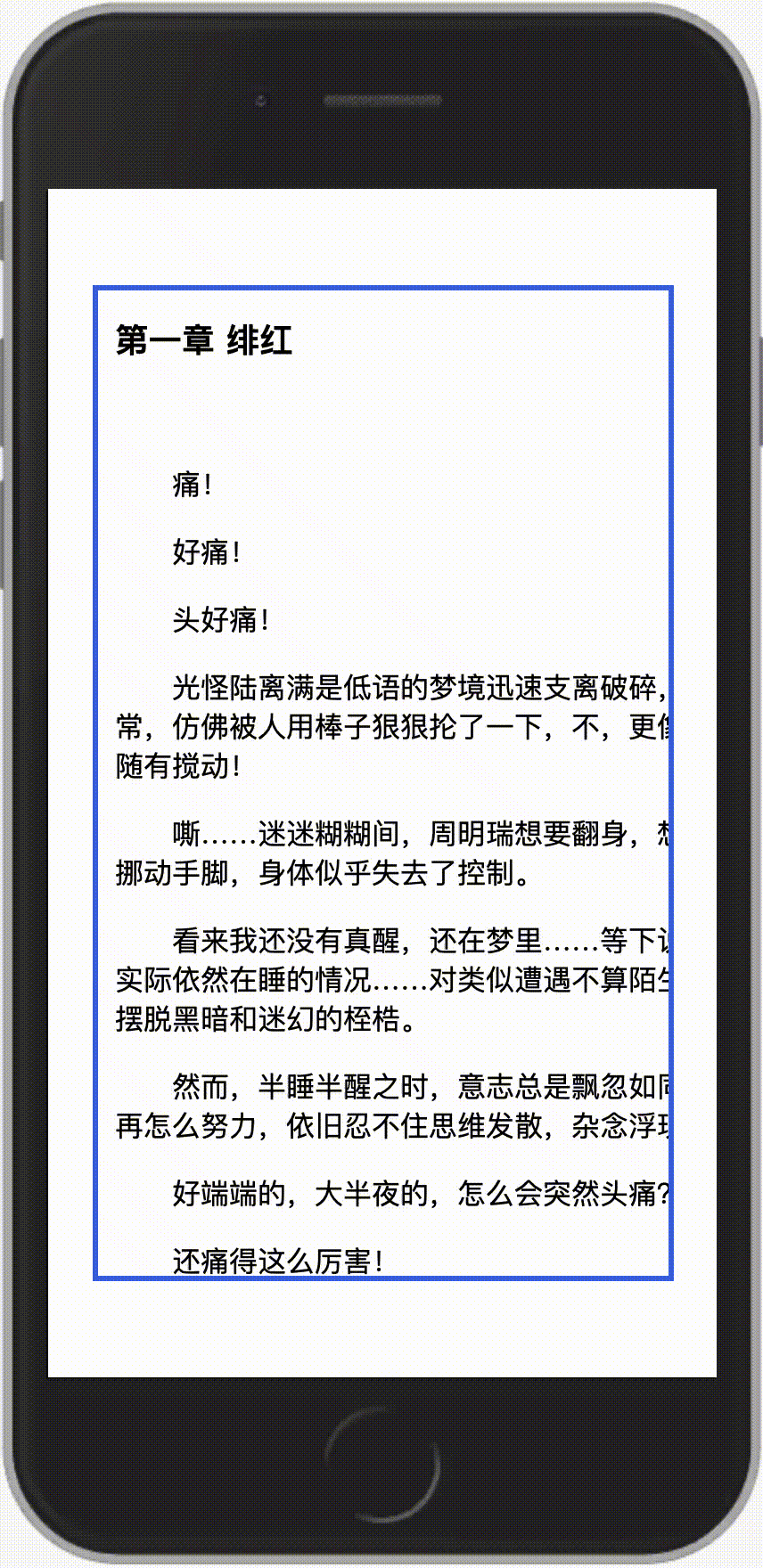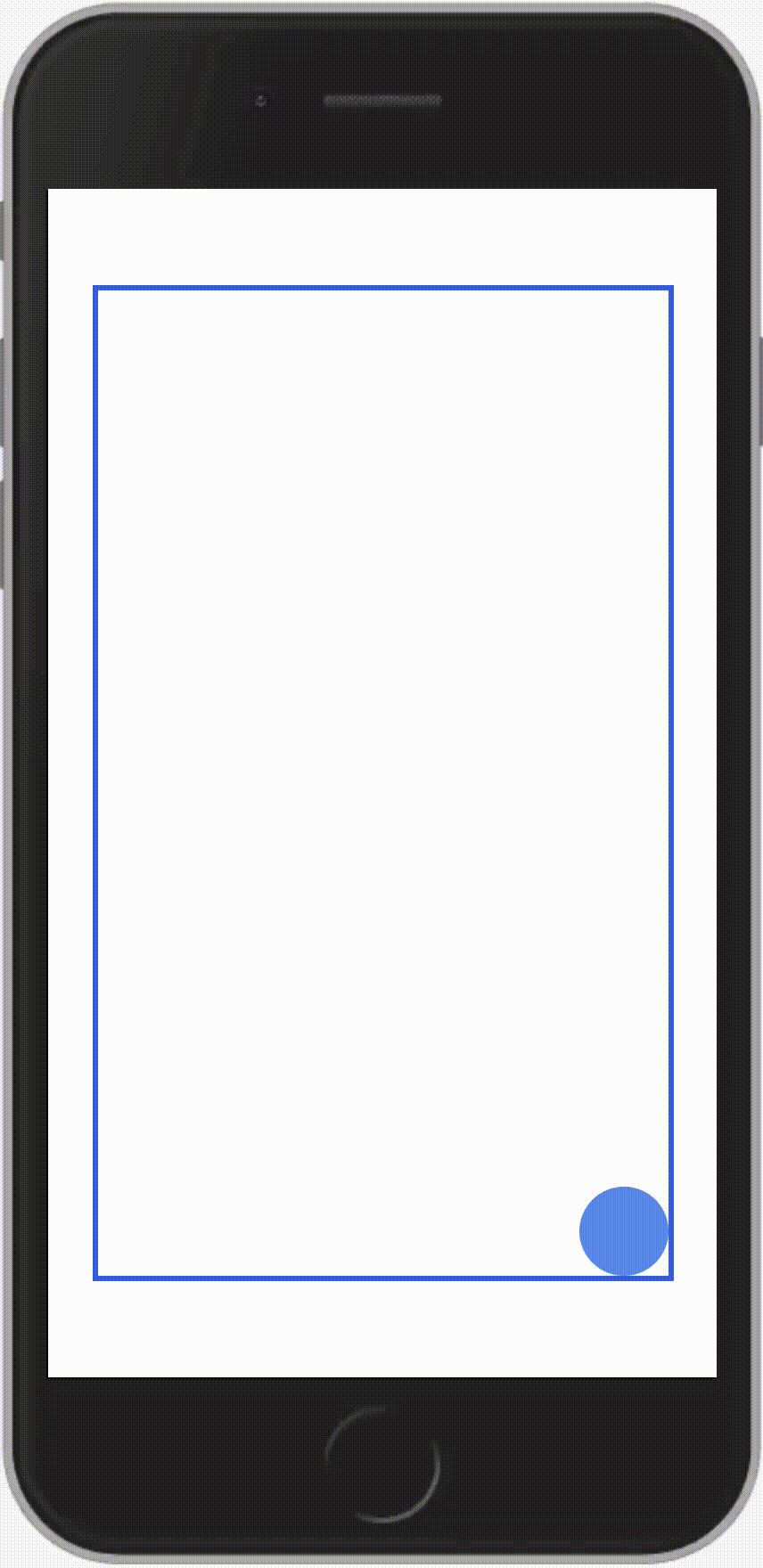## Vue3 的新语法

``````<template>
<h1>{{ color }}</h1>
</template>

<script>
export default {
data () {
return {
color: 'red'
}
}
}
</script>

<style>
h1 {
color: this.color;
}
</style>
``````

``````<template>
<h1>{{ color }}</h1>
</template>

<script>
export default {
data () {
return {
color: 'yellow'
}
}
}
</script>

<style>
h1 {
color: v-bind(color)
}
</style>
``````

`this.color` 发生变化时，`css` 也会一同做出响应。

## 九宫格面试题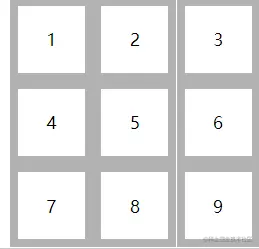## 你不知道的 CSS 负值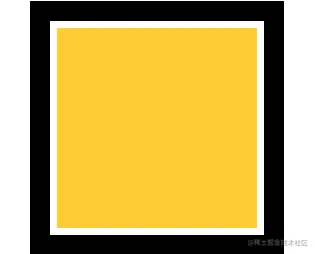``````<template>
<div></div>
</template>

<style>
div {
width: 200px;
height: 200px;
outline: 20px solid #000;
outline-offset: -118px;
}
</style>
``````

# 题目

## 情景再现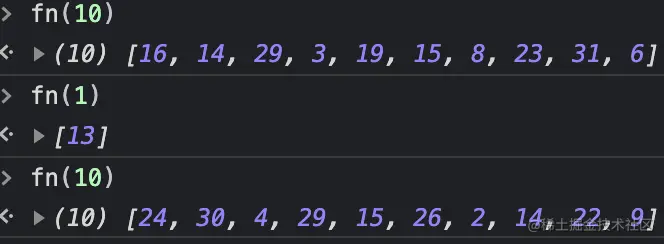``````const fn = num => {
let arr = []

for (let i = num; i-- > 0;) {
arr.push(Math.round(Math.random() * 30 + 2))
}

return arr
}
``````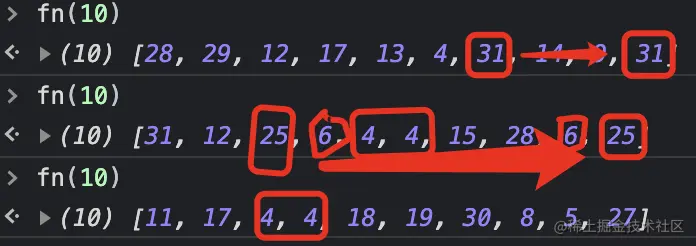``````const fn = num => {
let arr = []

for (let i = num; i-- > 0;) {
arr.push(Math.round(Math.random() * 30 + 2))
}

arr = [...new Set(arr)]

return arr
}
``````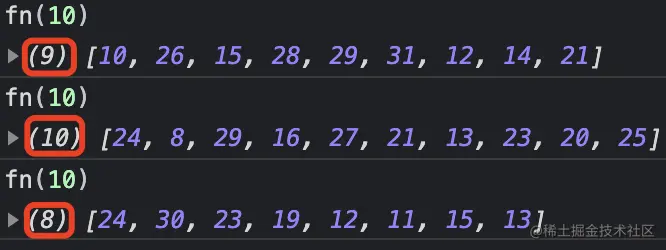``````const fn = num => {
let arr = []

for (let i = num; i-- > 0;) {
arr.push(Math.round(Math.random() * 30 + 2))
}

arr = [...new Set(arr)]

let len = arr.length

while (num - len > 0) {
arr = [...new Set(arr.concat(fn(num - len)))]
len = arr.length
}

return arr
}
``````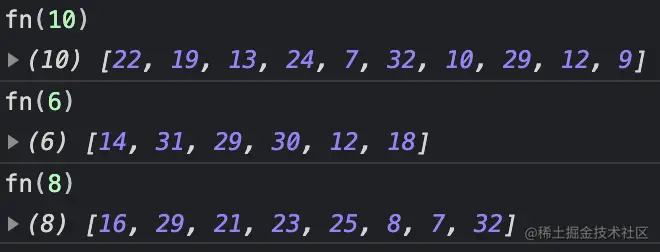# 二面加难度后的题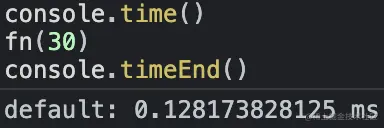0.1 毫秒，也还行啊！可能是数字小了吧，如果是生成从 0 ～ 10000 之间的随机数应该就崩溃了吧？修改一下函数：

``````const fn = num => {
let arr = []

for (let i = num; i-- > 0;) {
arr.push(Math.round(Math.random() * 10000))
}

arr = [...new Set(arr)]

let len = arr.length

while (num - len > 0) {
arr = [...new Set(arr.concat(fn(num - len)))]
len = arr.length
}

return arr
}
``````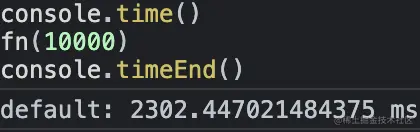``````const fn = num => {
const allNums = Array.from({ length: 31 }, (_, i) => i + 2)
const result = []

for (let i = num; i-- > 0;) {
result.push(allNums.splice(Math.floor(Math.random() * allNums.length), 1))
}

return result
}
``````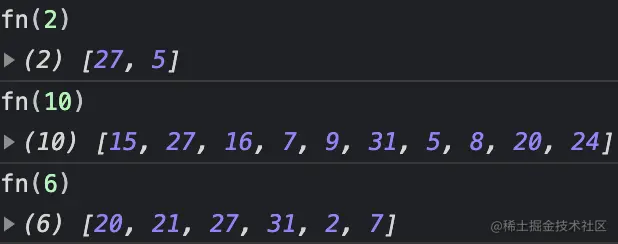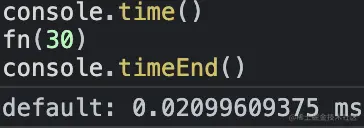``````const fn = num => {
const allNums = Array.from({ length: 10001 }, (_, i) => i)
const result = []

for (let i = num; i-- > 0;) {
result.push(allNums.splice(Math.floor(Math.random() * allNums.length), 1))
}

return result
}
``````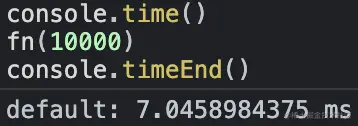``````const fn = (len, from = 0, to = 100) => {
const allNums = Array.from({ length: to - from }, (_, i) => i + from)
const result = []

for (let i = len; i-- > 0;) {
result.push(allNums.splice(Math.floor(Math.random() * allNums.length), 1))
}

return result
}
``````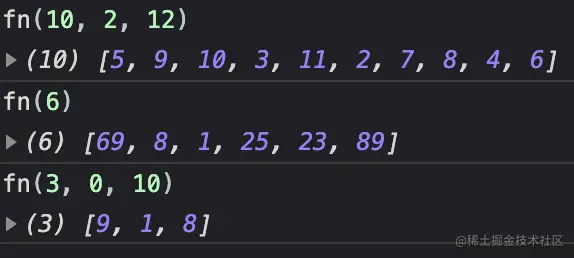``````'0123456'.substring(1, 5)
``````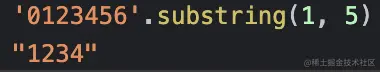``````const fn = (len, from = 0, to = 100) => {
const ratio = (to - from) / len
let result = []

if (ratio > 0.3) {
const allNums = Array.from({ length: to - from }, (_, i) => i + from)

for (let i = len; i-- > 0;) {
result.push(allNums.splice(Math.floor(Math.random() * allNums.length), 1))
}
} else {
for (let i = len; i-- > 0;) {
result.push(Math.round(Math.random() * to + from))
}

result = [...new Set(result)]

let length = result.length

while (len - length > 0) {
result = [...new Set(result.concat(fn(len - length, from, to)))]
length = result.length
}
}

return result
}
``````

# 用处``````const arr = ['题', '题', '题', ...'题']

const result = arr.filter(() => Math.random() > 0.5)

result.length = 10
``````

``````const arr = [0,1,2,3,4,5,6,7,8,9,10,11,12,13,14,15,16,17,18,19,20]

const fn = num => {
const result = arr.filter(() => Math.random() > 0.5)
result.length = num
return result
}
``````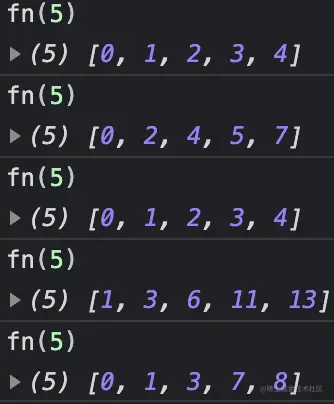# 往期精彩文章

••••••••••